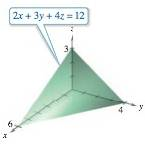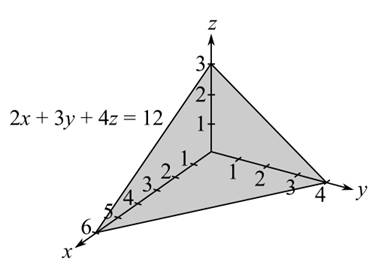Chapter 14.2, Problem 23E

Chapter
Section
Textbook Problem

Finding VolumeIn Exercises 21–26, use a double integral to find the volume of the indicated solid.To determine

To calculate: The volume of the solid given below.Explanation

Given:

The provided solid is:

Formula used:

The volume of a solid is provided by,

V=Rf(x,y)dA where, f(x,y)=z

Calculation:

The volume of a solid is provided by,

V=Rf(x,y)dA where, f(x,y)=z

From the figure,

f(x,y)=z=14(122x3y)

Now, for the x-y plane, put z=0, and get the following,

2x+3y=12

The provided diagram is projected on x-y plane as,

Or,

y=13(122x)=23x+4

So, y goes from 0 to 13(122x) and x goes from 0 to 6

Still sussing out bartleby?

Check out a sample textbook solution.

See a sample solution

The Solution to Your Study Problems

Bartleby provides explanations to thousands of textbook problems written by our experts, many with advanced degrees!

Get Started

Find more solutions based on key concepts